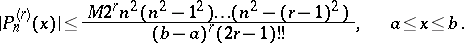# Markov inequality

Jump to: navigation, search

for derivatives of algebraic polynomials

An equality giving an estimate of the uniform norm of the derivative in terms of the uniform norm of the polynomial itself. Letbe an algebraic polynomial of degree not exceedingand let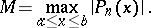Then for anyin,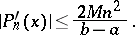(*)

Inequality (*) was obtained by A.A. Markov in 1889 (see ). The Markov inequality is exact (best possible). Thus, for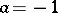,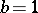, considering the Chebyshev polynomials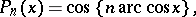then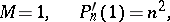and inequality (*) becomes an equality.

For derivatives of arbitrary order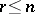, Markov's inequality implies that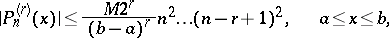which already for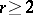is not exact. An exact inequality for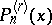was obtained by V.A. Markov :